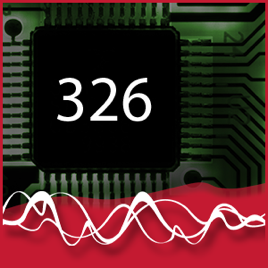12 afleveringen

This course provides an introduction to probabilistic models and methods for addressing uncertainty and variability in engineering applications with the aim of learning how to construct probability distributions and to calculate probabilities, expected values, and other statistics that are needed for practical problems. A balanced approach and coverage is provided for problems and applications on the one hand and mathematical and analytical models and methods on the other. Topics include fundamental concepts in probability theory (sample spaces, events, counting principles), conditional probability, independence, probability distributions, discrete and continuous random variables, probability density and cumulative distribution, functions of random variables, and random processes. Engineering applications of these concepts are chosen from areas such as communications, signal processing, electronics fabrication, and computer engineering. This course builds on calculus concepts from APSC 171.# ELEC 326 Probability and Random Processes Queen's University

• Technologie

This course provides an introduction to probabilistic models and methods for addressing uncertainty and variability in engineering applications with the aim of learning how to construct probability distributions and to calculate probabilities, expected values, and other statistics that are needed for practical problems. A balanced approach and coverage is provided for problems and applications on the one hand and mathematical and analytical models and methods on the other. Topics include fundamental concepts in probability theory (sample spaces, events, counting principles), conditional probability, independence, probability distributions, discrete and continuous random variables, probability density and cumulative distribution, functions of random variables, and random processes. Engineering applications of these concepts are chosen from areas such as communications, signal processing, electronics fabrication, and computer engineering. This course builds on calculus concepts from APSC 171.